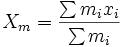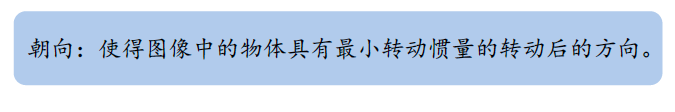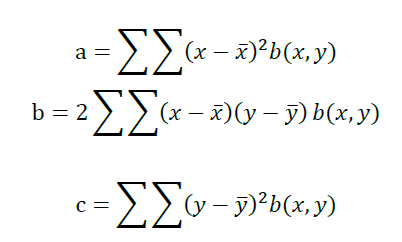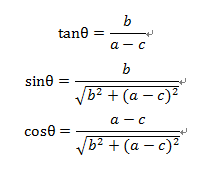# [图像] 二值图像的位置、朝向与投影

二值图像就是由0,1组成的图像。我们可以通过一些简单的计算获取图像的一系列信息。

面积：

所有1的总和

位置：

图像的位置一般由质心来表达，我们可以直接根据质心的定义来计算x轴方向和y轴方向的质心，然后得到中心(xm,ym)朝向：

《机器视觉》一书给出的朝向的定义：在这里，我们需要求的事最小二阶矩所对应的轴。

我们用一个点和直线与x轴的夹角来定义朝向所在的轴位置，首先我们经过推导可以得到：最小二阶矩对应的轴经过质心。

接下来，我们只需求出夹角。其中：投影:

简单而言，投影就是某个方向上，1的总和。

在具体实现中，我们可以使用增量的思想来加速运算。我们知道在显示加载图像的时候，我们是用扫描线一行一行地加载图像的，所以我们可以在这个过程中计算一阶矩和二阶矩，进而计算二值图像的一些特征的值。

matlab代码：

function twoValueImg(f)
[m,n] = size(f);
s = 0; %面积
x1 = 0; % 一阶矩 x方向
y1 = 0; % 一阶矩 y方向
a = 0; % 二阶矩
b = 0; % 二阶矩
c = 0; % 二阶矩
for i = 1:m
for j = 1:n
if(f(i,j)==1) % 增量思想
s = s + 1;
x1 = x1 + i;
y1 = y1 + j;
a = a + i*i;
b = b + i*j;
c = c + j*j;
end
end
end